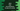# Java program to find the circumference and area of a circle### Java program to find circumference and area of a circle :

In this tutorial, we will learn how to find the area and circumference of a circle using Java. We only need the radius of the circle to find both of these values. Area is _‘PIradiusradius’_and circumference is ‘2PIradius’. Let’s take a look how to calculate these values :

### Java Program :

``````import java.util.Scanner;

public class Main {

/**
* Utility function for System.out.println
*
* @param message : string to print
*/
private static void println(String message) {
System.out.println(message);
}

/**
* Utility function for System.out.print
*
* @param message : string to print
*/
private static void print(String message) {
System.out.print(message);
}

/**
* main method
*
* @throws java.lang.Exception
*/
public static void main(String[] args) throws java.lang.Exception {
//1

//2
Scanner scanner = new Scanner(System.in);

//3
print("Enter the radius of the circle : ");

//4
circumference = 2 * Math.PI * radius;

//5
System.out.printf("Area of the circle : %.2f", area);
println("");
System.out.printf("Circumference of the circle :%.2f ", circumference);

}

}
``````

### Sample Output :

``````Enter the radius of the circle : 100
Area of the circle : 31415.93
Circumference of the circle :628.32

Enter the radius of the circle : 19.4
Area of the circle : 1182.37
Circumference of the circle :121.89

Enter the radius of the circle : 10
Area of the circle : 314.16
Circumference of the circle :62.83
``````

### Explanation :

1. Create three ’double’ variables to store the radius, area and circumference of the circle.

2. Create one ’Scanner’ object to take the inputs from the user.